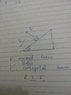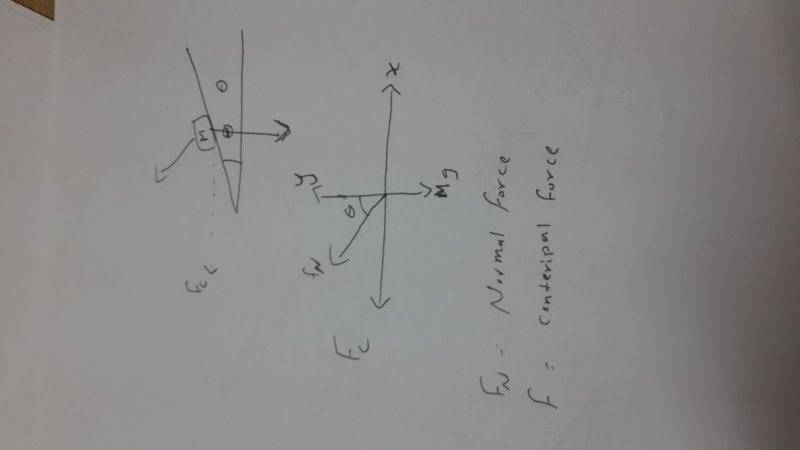# Bank Curve Problem: Normal Force on Moving Car

• oreo
In summary, the normal force and weight are two components of the centripetal force on a moving object on a track. The centripetal force is directed towards the center of the circle while the normal force is perpendicular to it. The direction of the normal force can be determined by drawing a free body diagram of the problem.

#### oreo

Is normal force on moving car on a track a component of weight or vice versa.

Because the centripetal force is aiming in the direction of x-axis ,, The Normal Force would be resolved into to components one is equal to the weight of the moving object

It means that weight is component of normal force. Is centripetal force directed towards center of circle or towards the axis because these are two different cases.

If you draw a free body diagram of this problem what would you have ,,

the Mg lies on the negative y-axis pointing toward the center of Earth , the centripetal force is pointing toward either positive x-axis or negative x-axis [horizontal plane] , And the normal force would have an angel with the x-axis [which will be resolved into components]

Maged Saeed said:
If you draw a free body diagram of this problem what would you have ,,

the Mg lies on the negative y-axis pointing toward the center of Earth , the centripetal force is pointing toward either positive x-axis or negative x-axis [horizontal plane] , And the normal force would have an angel with the x-axis [which will be resolved into components]
I want to ask that is centripetal force acting towards the center of circular track or towards its central axis(x direction)

To the center of the circle NOT to the center of the x-direction ,, I didn't mean that ,,

:D

Maged Saeed said:
To the center of the circle NOT to the center of the x-direction ,, I didn't mean that ,,

:D
If it is then the normal force is perpendicular to the track ( ie perpendicular to centripetal force) then it would have no component providing the requisite force. Here is the figure in photo.

#### Attachments

•14203180279401100976962.jpg
27.6 KB · Views: 434
Actually what you have drawn is somehow mistaken , The centripetal force direction is a horizontal line from the moving object to the center of the circular track ,,
See this picture ,,Maged Saeed said:
Actually what you have drawn is somehow mistaken , The centripetal force direction is a horizontal line from the moving object to the center of the circular track ,,
See this picture ,,
View attachment 77201
That was I wanted to confirm
You had first said that it is towards center but if it is so then the normal force would be at right angle to centripetal force as I drew but the figure you drew confirms that it is towards the axis. I took direction of normal force as y- axis.

Okay ,, The picture implies my point And sorry for my English
(:

Maged Saeed said:
Okay ,, The picture implies my point And sorry for my English
:)
Thank you for helping me. We done it together.

•Maged Saeed

## What is the Bank Curve Problem?

The Bank Curve Problem is a physics concept that involves a car or any other object moving in a circular path on a banked curve. It deals with the forces acting on the car and how it maintains its trajectory on the curve.

## What is the normal force on a moving car on a banked curve?

The normal force is the force that acts perpendicular to the surface of the banked curve. It is responsible for keeping the car from sliding down the curve and maintaining its circular motion. The magnitude of the normal force increases with the angle of the bank and the speed of the car.

## How does the angle of the bank affect the normal force on a moving car?

The angle of the bank directly affects the magnitude of the normal force. As the angle of the bank increases, the normal force also increases. This is because a steeper bank provides more support for the car to maintain its circular path.

## What happens to the normal force if the car is moving at a constant speed?

If the car is moving at a constant speed, the normal force remains constant as well. This is because the centripetal force required to keep the car in its circular path is balanced by the normal force acting on the car.

## What other factors can affect the normal force on a moving car on a banked curve?

The normal force can also be affected by the mass of the car and the radius of the banked curve. A heavier car will require a greater normal force to maintain its circular path, while a smaller radius curve will also require a greater normal force.